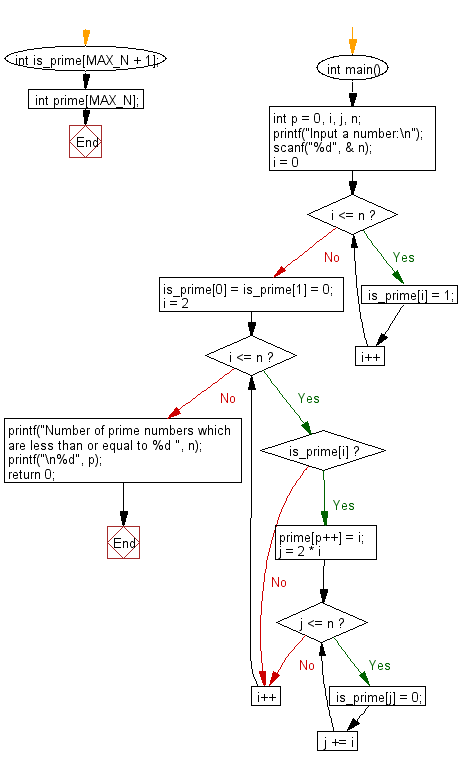﻿ C : Prime numbers that are less than or equal to a integer

# C Exercises: Find the prime numbers which are less than or equal to a given integer

## C Basic Declarations and Expressions: Exercise-136 with Solution

Write a C program to find prime numbers that are less than or equal to a given integer.

Input:
n (1 <= n <= 999,999)

Sample Solution:

C Code:

``````#include<stdio.h>

#define MAX_N 999999
int is_prime[MAX_N + 1];
int prime[MAX_N];

int main() {
int p = 0, i, j, n;

// Prompting the user to input a number
printf("Input a number:\n");
scanf("%d", &n);

// Initializing the is_prime array to all true (1)
for (i = 0; i <= n; i++)
is_prime[i] = 1;
is_prime = is_prime = 0; // 0 and 1 are not prime

// Finding prime numbers using the Sieve of Eratosthenes algorithm
for (i = 2; i <= n; i++) {
if (is_prime[i]) {
prime[p++] = i; // Storing prime numbers in the prime array
for (j = 2 * i; j <= n; j += i)
is_prime[j] = 0; // Marking multiples of a prime as not prime
}
}

// Printing the number of prime numbers and the count
printf("Number of prime numbers which are less than or equal to %d ", n);
printf("\n%d", p);

return 0; // End of the program
}
``````

Sample Output:

```Input a number:
123
Number of prime numbers which are less than or equal to 123
30
```

Flowchart:C programming Code Editor:

What is the difficulty level of this exercise?

Test your Programming skills with w3resource's quiz.

﻿